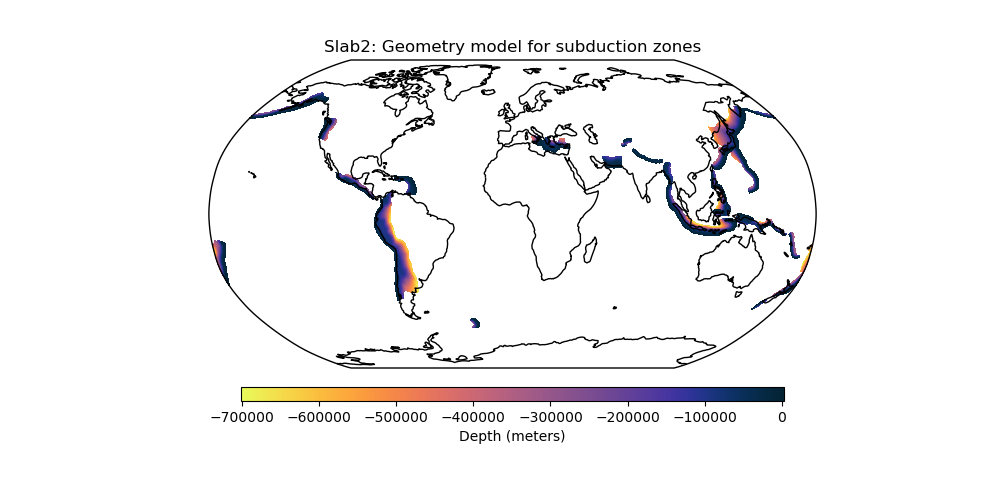# Slab2 - A Comprehensive Subduction Zone Geometry Model¶

Slab2 is a three-dimensional compilation of global subduction geometries, separated into regional models for each major subduction zone. More information at the USGS website.import rockhound as rh
import matplotlib.pyplot as plt
import cmocean
import cartopy.crs as ccrs

from rockhound.slab2 import ZONES

# Fetch all Slab2 subduction zones and add them inside a list
subduction_zones = []
for zone in ZONES:
subduction_zones.append(rh.fetch_slab2(zone))

# Get min and max values of the subducting plates' depths
vmax = max([grid.depth.actual_range for grid in subduction_zones])
vmin = min([grid.depth.actual_range for grid in subduction_zones])

# Plot the depth of each subducting plate inside Slab2 with the same colorscale
plt.figure(figsize=(10, 5))
ax = plt.axes(projection=ccrs.Robinson())
for grid in subduction_zones:
pc = grid.depth.plot.pcolormesh(
cmap=cmocean.cm.thermal_r,
ax=ax,
transform=ccrs.PlateCarree(),
vmin=vmin,
vmax=vmax,
)
ax.set_title("Slab2: Geometry model for subduction zones")
plt.colorbar(
pc,
ax=ax,
label="Depth (meters)",